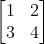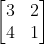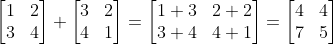# Program for addition of two matrix in c++

Today we are going to discuss a very frequently asked programming question. program for addition of two matrix in c++. It is a school level as well as a college level coding question. In addition, it is one of the basic must know programs.

Without much delay, let’s get our coding brains to use and get started!

## A Little Background on Matrices:

We can define matrices as rectangular arrays of numbers, symbols or expressions arranged in rows and columns.

Hence, some of the key points related to matrices:

1. A matrix has m rows and n columns.
2. It is represented by m x n or m-by-n.
3. m and n are called the dimensions of a matrix.
4. Additionally, one can use matrices to work with linear equations, i.e. systems of linear equations in a compact manner.
5. While solving linear transformations, we use matrix multiplications. It is also called a linear map.

However, if we need to know about matrices, we have to be aware of some terms related to them. This will also help the further program explanations.

1. Element : these are the individual item in a matrix.
2. Row Vector : A single row matrix.
3. Column Vector : A single column matrix.
4. Square Matrix : A matrix which has the same number of rows and columns.

Below is the visual representation of a Matrix:Usually, we use matrices to represent systems or list data. We can perform operations of matrices because their entries are numbers. Then, we add the matrices by adding the respective entries.

However, there is only one condition, i.e. the entries must correspond to each other. Hence, addition of matrices is only possible when two or more matrices have similar dimensions.

There are two properties involved with the addition of matrices. And, they are both commutative and associative. In order to understand, let’s say:

For instance, we have two matrices A and B. A+B = B+A. This is the commutative nature.

As for the associative property, let there be three matrices A,B and C. Associativity of addition of matrices means that, (A+B)+C= A+(B+C).

You can best understand the addition of matrices by a proper example. So, here it is:

Let A and B be matrices such that A=and B =.

Then, A + B =As you can see, Matrix addition is very simple to do and is very useful.

## C++ Programs for Matrix Addition:

Now, there are many ways to implement a C++ program for matrix addition. We can use a function, classes, constructors, etc. The simple method is also important and useful. In this post, we are going to learn three major methods for coding the program for matrix addition.

Simple addition of matrices will not include any complex addition of functions or classes. It will just be a program taking user input and displaying the desired result.

#### Algorithm:

This is the simplest method of addition of matrices. But before you jump into the code make sure to go through the algorithm and try coding yourself.

Without further delay, let us get right to the algorithm for simple addition of matrix with C++

1. Start
2. Define rows and column variable.
3. Next, we define array variables for two matrices as m1, m2 and also one for sum.
4. Ask the user to enter the number of rows and column with the limit in between 1 and 10.
5. Using for loop, take the elements entry from the user according to the number of rows and columns.
6. Then, calculate the sum of two matrices using two for loops nested to each other.
7. Finally, display the output of the program.
8. Stop.

#### Program:

``//C++ program for simple addition of Matrices. #include<iostream>using namespace std;int main(){   int row, col, m1, m2, sum;   //Defining the matrices by entering rows, columns.   cout<<"Enter the number of rows(should be >1 and <10): ";   cin>>row;   cout<<"Enter the number of column(should be >1 and <10): ";   cin>>col;   cout << "Enter the elements of first matrix(enter first row first): ";   for (int i = 0;i<row;i++ ) {     for (int j = 0;j < col;j++ ) {       cin>>m1[i][j];     }   }   //Entering elements by the user   cout << "Enter the elements of second matrix(enter first row first): ";   for (int i = 0;i<row;i++ ) {     for (int j = 0;j<col;j++ ) {       cin>>m2[i][j];     }   }//Calculating sum    for (int i = 0;i<row;i++ ) {      for (int j = 0;j<col;j++ ) {        sum[i][j]=m1[i][j]+m2[i][j];      }   }   //Displaying output      cout<<"Output: ";      cout<<endl;      for(int i = 0; i < row; ++i)        for(int j = 0; j < col; ++j)        {            cout << sum[i][j] << "  ";            if(j == col - 1)            cout << endl;        }   return 0;}``

#### Program Explanation:

The explanation for the simple addition method is simple, just like its name. To draw you an outline, we take user inputs.

Then, we use the required amount of for loops to enter the matrices and calculate their sum. In the end, we display the output, which is the sum of these matrices.

#### Output:

The program we just executed gives the following output:

### C++ Program for Matrix Addition using Class and Functions:

As the name suggests, in the C++ Program for Matrix addition using classes and functions, we will calculate the sum of matrices using classes and functions.

This is a little more complex than the previous version. However, this is more useful when it comes to large programs where we involve multiple classes and functions.

Additionally, in this program, we will define a user defined class and a function to complete our calculations. Hence, it is a very interesting approach and will get your coding brains to work. Let us start with the algorithm as always.

#### Algorithm:

The Algorithm for the C++ Program for Matrix addition will make you closely follow what procedure we followed while coding. It will provide the perfect overview of the program so that you can code without even looking at the program.

1. Start
2. Define a class Add. Member functions : sum(int r, int c), type void.
3. Inside member function void sum,
4. Define m1, m2 and s for two arrays and sum respectively.
5. Then, enter the elements using a for loop.
6. Calculate the sum using a loop.
7. Create an output loop using a for loop.
8. Define main function.
9. Take input from the user for rows and columns.
10. Create class object obj.
11. Then call the sum function through this class object.
12. Finally, display the result.
13. Stop.

#### Program:

``//C++ Program for Matrix addition using classes and function#include<iostream>using namespace std;//Defining Class Add for additionclass Add{  public:  //sum function for entering the elements and calculating sum    void sum(int r, int c){      int m1[r][c], m2[r][c], s[r][c];      cout << "Enter the elements of  first matrix: ";      for (int i = 0;i<r;i++ ) {        for (int j = 0;j < c;j++ ) {          cin>>m1[i][j];        }      }      cout << "Enter the elements of second matrix: ";      for (int i = 0;i<r;i++ ) {        for (int j = 0;j<c;j++ ) {          cin>>m2[i][j];        }      }      for (int i = 0;i<r;i++ ) {        for (int j = 0;j<c;j++ ) {          s[i][j]=m1[i][j]+m2[i][j];        }      }     //Displaying output      cout<<"Output: ";      cout<<endl;      for(int i = 0; i < r; ++i)        for(int j = 0; j < c; ++j)        {            cout << s[i][j] << "  ";            if(j == c - 1)            cout << endl;        }     }};//main function for entering the number of rows and columns. And, calling class and functionint main(){   int row, col;   cout<<"Enter the number of rows(should be >1 and <10): ";   cin>>row;   cout<<"Enter the number of column(should be >1 and <10): ";   cin>>col;   Add obj;   obj.sum(row, col);   return 0;}``

#### Program Explanation:

I am sure you must have some doubts regarding this method. Don’t worry, this detailed program explanation will help you understand the program better.

First, we have defined a class named Add which will also have our function sum.

In the sum function, there are three variables initialized. m1 for the first array, m2 for the second array and s for the sum array.

Next, we ask the user to enter the elements of the first array. Then, we read the elements using a nested for loop structure.

Similarly, we enter and read the elements of the second array.

Like the simple method of matrix addition, we define a loop for adding these two arrays.

In the next step, we have created a display loop which is again similar to the display loop of the simple addition method.

Now, the main function is the most important section of this program.

In the main function, we first ask the user to enter the number of rows and column.

Next, We create a class object and name it as obj. We call the member function sum through this obj and then pass the values of rows and columns entered by the user.

Note: Objects are instances of a class. It is through them that we can access class methods and member variables.

Finally, when the compiler passes these values to the sum function, the rest of the loops gets executed and we get the result.

#### Output:

The program we just executed gives the following output:

### C++ Program for Matrix Addition using Functions:

This program follows the similar logic to the one discussed above, i.e the C++ Program for matrix addition using class and function. Here, we will include function only as a means to solve the addition of two matrices.

Therefore, to make the understanding more clear, we have listed the algorithm and a detailed program explanation below.

#### Algorithm:

You will notice that the algorithm will be a bit similar to the previous method. Because, we have only omitted one constraint, i.e. class from the program. The procedure of coding is basically the same.

Thus, I hope you realise it after going through the simple algorithm of C++ Program of Matrix addition using functions.

1. Start
2. Define a function sum(int, int) with two integer variables.
3. Then, define the main function with row and column variable.
4. Ask the user to enter the rows and columns for the matrices.
5. Then, Pass the value of row and column to the sum function.
6. In the sum function, declare m1, m2 and s variables for array 1, array 2 and sum array, respectively.
7. Then use nested for loops for entering elements into the array and adding them to the sum array.
8. Finally, create a display loop to print the required sum of matrices.
9. Stop.

#### Program:

``//C++ Program for matrix addition using functions#include<iostream>using namespace std;void sum(int, int);//main function for entering rows and columnsint main(){   int row, col;   cout<<"Enter the number of rows(should be >1 and <10): ";   cin>>row;   cout<<"Enter the number of column(should be >1 and <10): ";   cin>>col;   sum(row, col);   return 0;}//sum function to enter the elements of the matrix and calculate sumvoid sum(int r, int c){   int m1[r][c], m2[r][c], s[r][c];   cout << "Enter the elements of  first matrix: ";   for (int i = 0;i<r;i++ ) {     for (int j = 0;j < c;j++ ) {       cin>>m1[i][j];     }  }   cout << "Enter the elements of  second matrix: ";   for (int i = 0;i<r;i++ ) {     for (int j = 0;j<c;j++ ) {       cin>>m2[i][j];     }   }   for (int i = 0;i<r;i++ ) {      for (int j = 0;j<c;j++ ) {       s[i][j]=m1[i][j]+m2[i][j];     }   }    //Displaying output      cout<<"Output: ";      cout<<endl;      for(int i = 0; i < r; ++i){        for(int j = 0; j < c; ++j)        {            cout << s[i][j] << "  ";            if(j == c - 1)            cout << endl;        }     }}``

#### Program Explanation:

From the array and the code, you must be clear that this code is almost same as the previous one in which we used classes. However, it is very feasible as compared to both of the previous methods. Thus, we can use this block of code anywhere with ease without having to worry about many definitions.

Hence, to have another round of explanation and more clarity, let me give you one brief description of how we executed the above code.

At first we have just declared the function just above the main with two integer variables which will act as the formal parameters of rows and columns.

Next, we have defined the main function such that the user will enter the number of rows and column.

After that, we have passed these values or the actual parameters to the sum function.

Finally, for the sum function, we have declared two array variables and one sum array variable. We have followed the same method of looping like the other two methods to enter the matrices and add them to the sum array.

Similarly, we have defined the display loop to print the output.

#### Output:

The program we just executed gives the following output: